# How to Create a Dynamic Chart Range in Excel?

A Dynamic chart range is the range of a data set which automatically updates on any modifications in the original data set. It is beneficial because at some point in time we need to add or delete data from the original data set. So, we want a method to automatically update the chart on performing any modifications in the source data set. This is known as Dynamic Chart Range in which as the source data changes, the dynamic range updates, and within a fraction of seconds the chart associated with the data set automatically gets updated.

In this article, we are going to see how to create a dynamic chart range in Excel. Basically, there are two methods :

• Using the Excel Table made with the data set.
• Using the Formula method.

Let’s consider an example shown below and see how to create a dynamic chart range using the above-listed methods.

Example: Consider the data set shown below which consists of the data about the number of students enrolled in our famous courses. We will create a dynamic range so that if any new data are either added or deleted the chart gets modified automatically.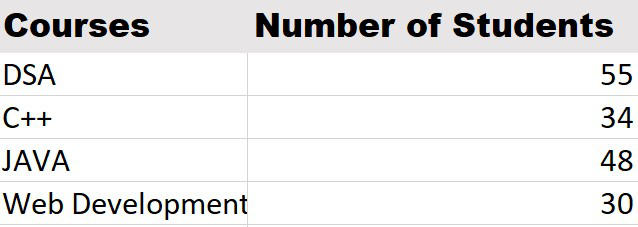## Dynamic Chart Range using the Excel Table:

This feature is available from Excel 2007 version and higher which we generally use nowadays. It is the most efficient method because when we add new data to the original source table it gets automatically updated.

The steps to create a dynamic chart range using a table are as follows :

Step 1: Select the table.

Step 2: Click on the Insert tab from the top of the Excel window.

Step 3: Click on the Table.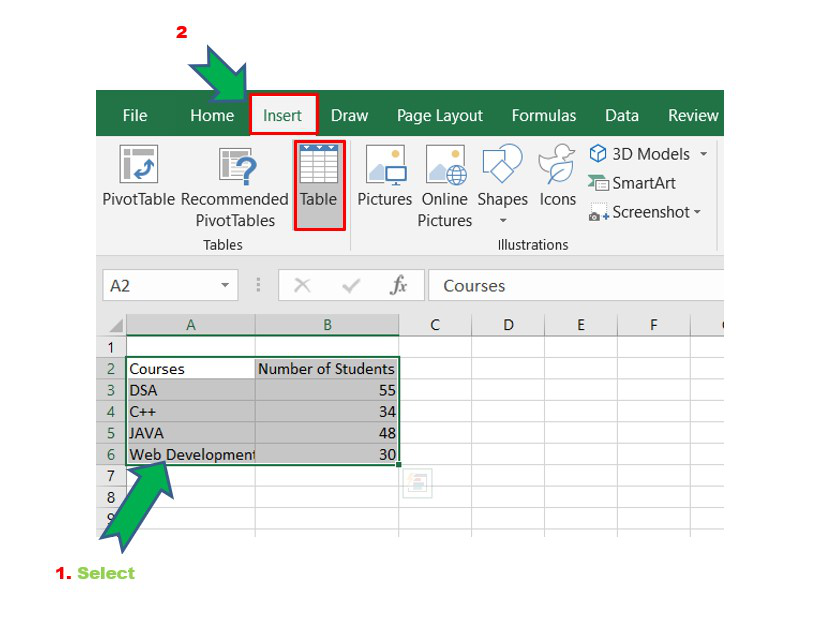Step 4: The Create Table window opens. Since the above table has headers “Courses”, “Number of Students” check the box as shown below and then click on OK.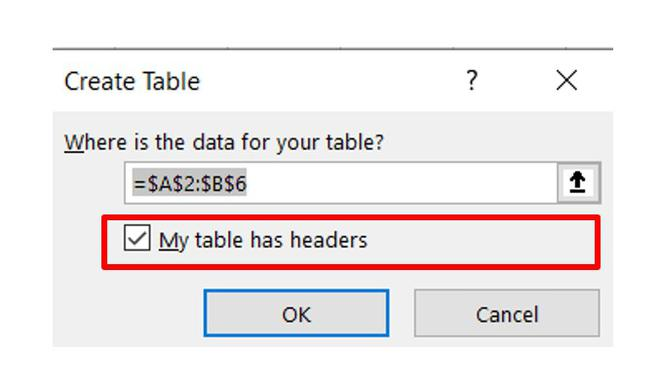`The shortcut to the above two steps is CTRL+T which will open the Create Table window directly.`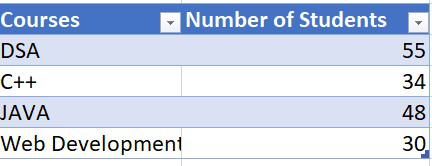Dynamic Range Excel Table

Step 5: Now select the entire table and go to Insert and from the Chart Group sets select the 2-D column. You can choose any chart as per requirements.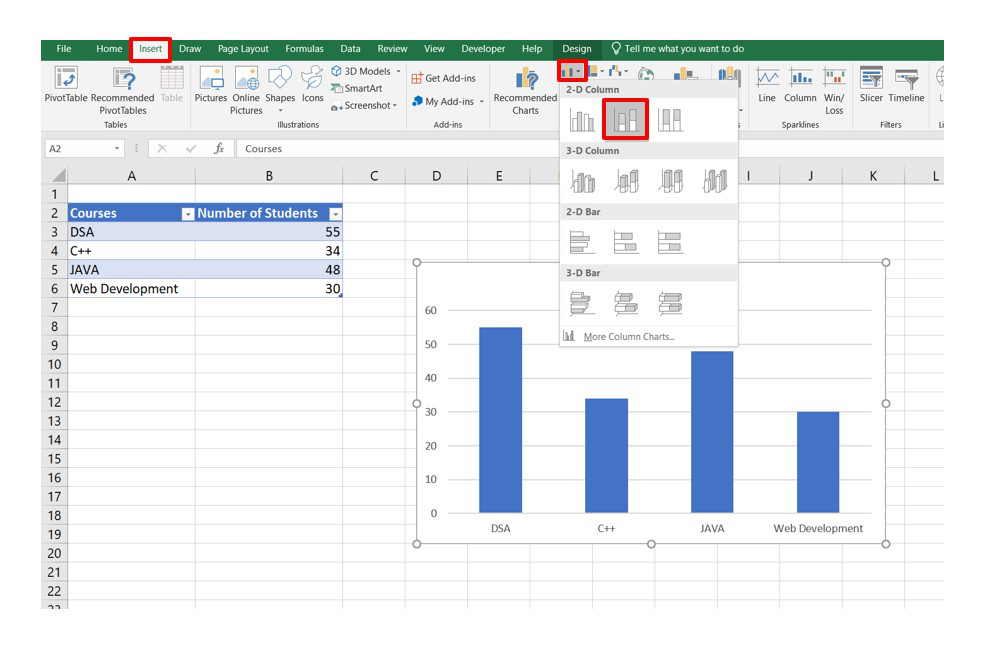Step 6: Now we insert new data in the Excel table and observe what happens in the chart.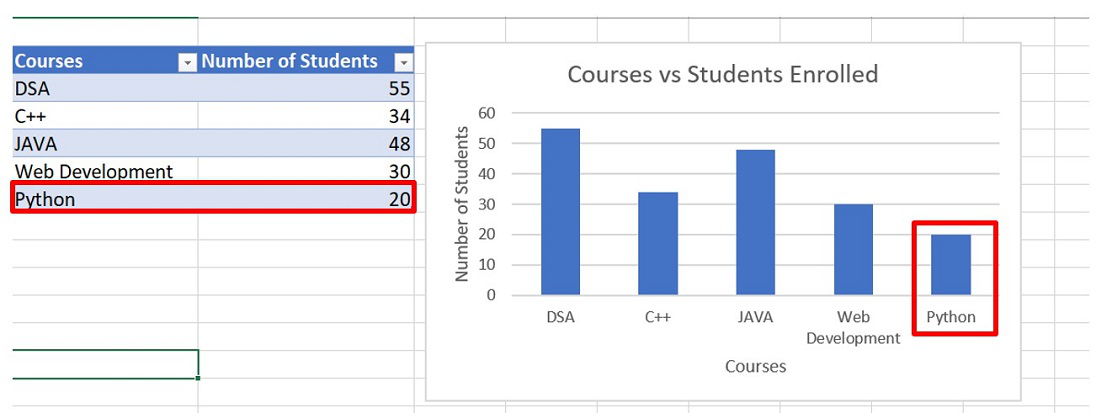It can be observed that as we enter the new data the chart gets automatically updated.

## Using the Excel Formula:

It is an alternate method that can be used in any version of Excel. The functions used for generating formulas are “OFFSET”, “COUNTIF”.

OFFSET: It is basically used to create a reference offset from a starting point. To create a dynamic range we need the OFFSET function.

Syntax:

```= OFFSET(reference,rows,cols,[height],[width])

arguments : reference,rows,cols,[height],[width]```

COUNTIF : It is basically used to count cells that match the criteria or a single condition. In criteria, we use LOGICAL OPERATORS like (<,>,>=,<=,<>) and wildcards like (*,?) in case of any partial matching.

Syntax:

```= COUNTIF(range,criteria)

arguments : range,criteria```

Now, let’s discuss the key steps to be followed to create a dynamic range chart.

Step 1 : Select any cell in Excel and write the formula as shown below for both “Courses” and “Number of Students”. Copy this formula and store it somewhere, probably a notepad as we need it again.

The cell range is taken from Row 3 to Row 102 which is 100 cells in total. So, we created a dynamic range for the user to enter new data into the existing data set.

For example : For “The Number of Students” column the cell range will be from B3 to B102.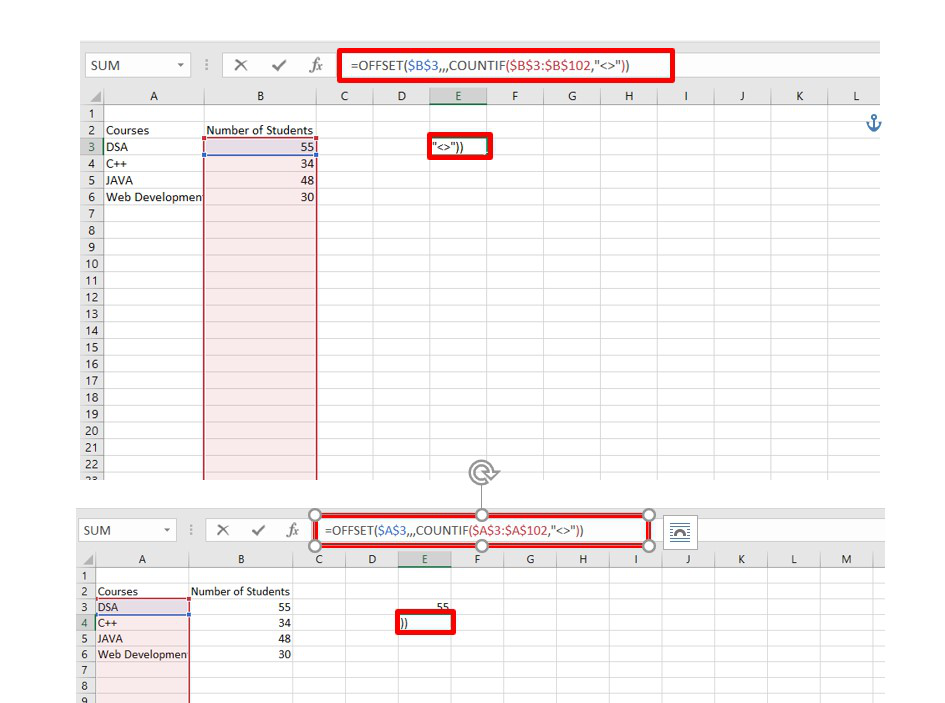Step 2: Now go to the Formulas tab and select Name Manager.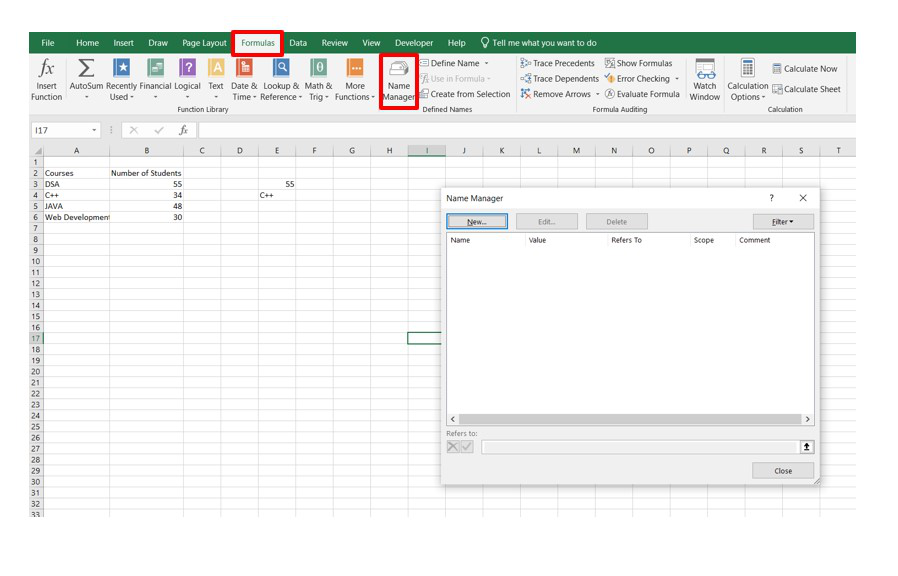Step 3: Now specify a new name in the Name Manager window.

In Refers to: Copy paste the previously written formula for the Number of Students column and click OK.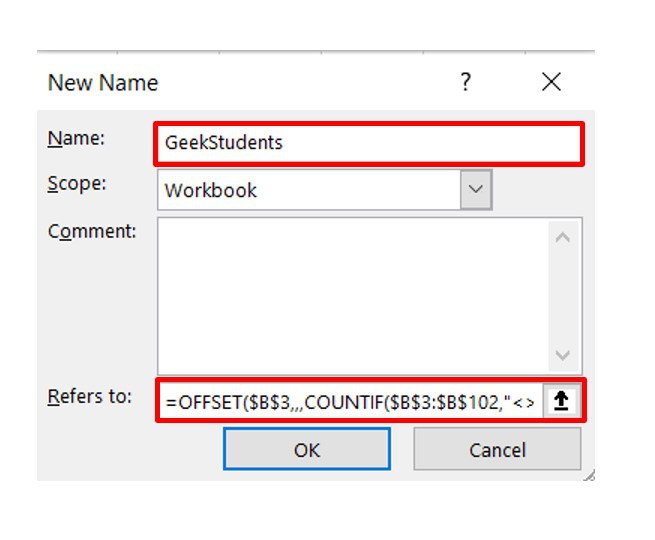Similarly, do it for the “Courses” column by providing a new name GeekCourses.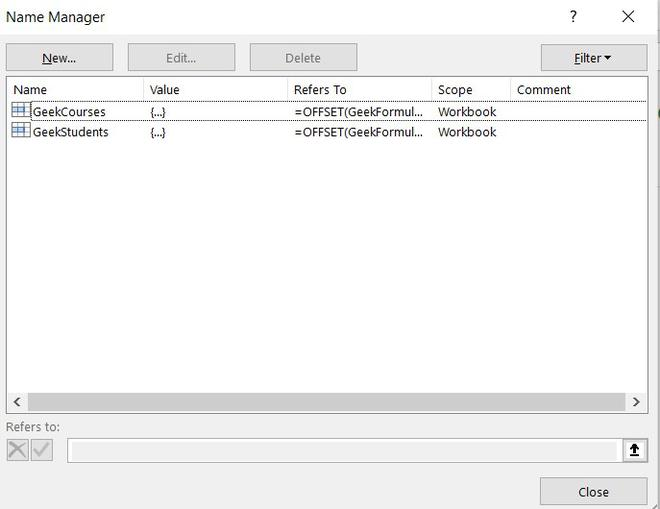In this step basically, we are creating two new ranges GeekCourses and GeekStudents which refer to the original data set values. Now, if we add any new data in the previous data set it will automatically be updated in the ranges created in this step.

Step 4 : Now we will create a new dynamic chart associated with the Dynamic Range created using the formulas in the above step.

Insert a blank chart and then go to the Design tab and click on Select Data.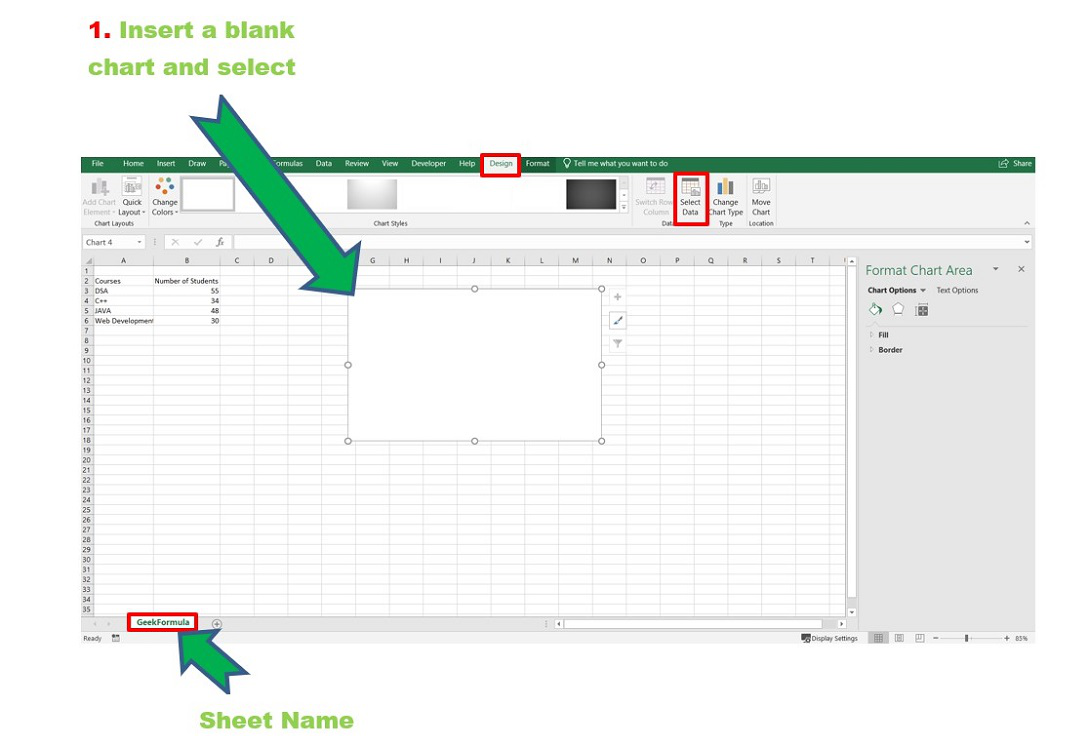Step 3: The Select Data Source dialog box opens. Now click on Add.

In the Series Value enter the following command :

```Sheet_Name!(Name_Ranged_Formula)

Name_Ranged_Formula : The dynamic range created using the Formula.```

In our case it is :

`GeekFormula!GeekStudents`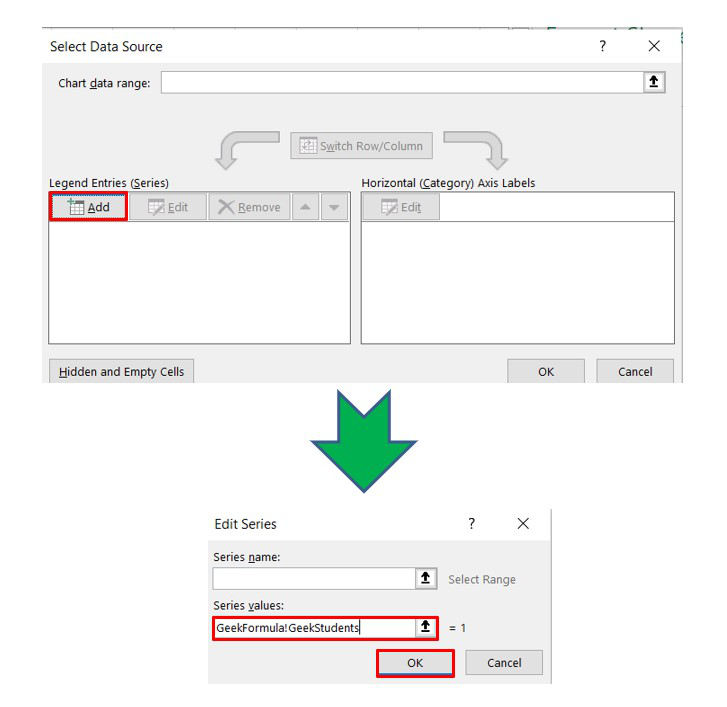Now click OK. We can observe that the blank chart is now updated with the data set values. However, the Horizontal-axis (for Courses) is not yet correct. For that, we need go to the Edit tab and write the axis label range as :

`GeekFormula!GeekCourses`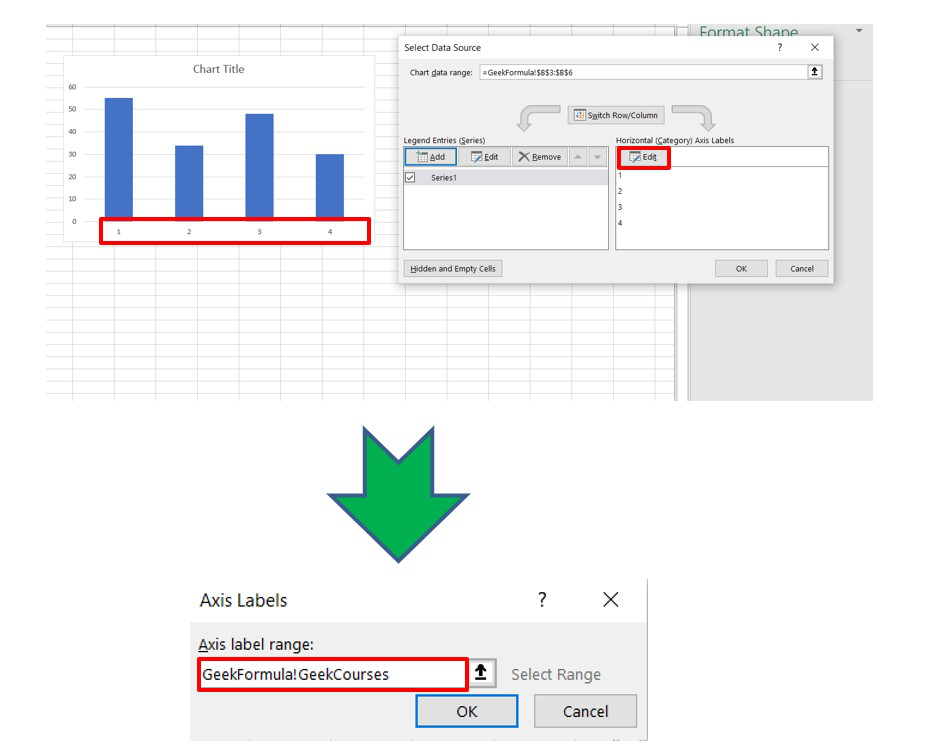Click OK. The Dynamic chart is now ready.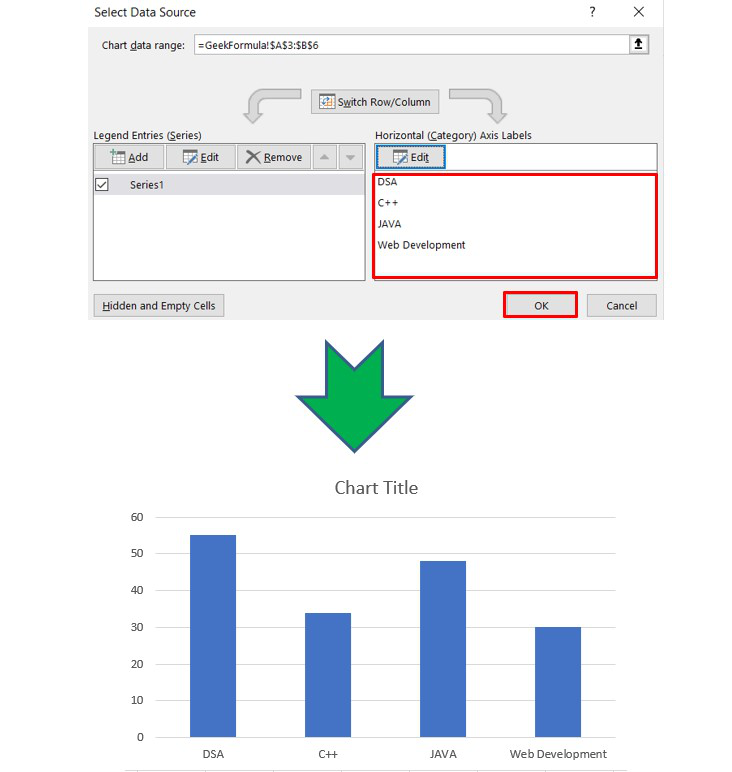Now, enter new data in the original data set and it can be observed that the chart automatically updates. Also, if you delete any data the chart will delete those entries and modifies itself automatically.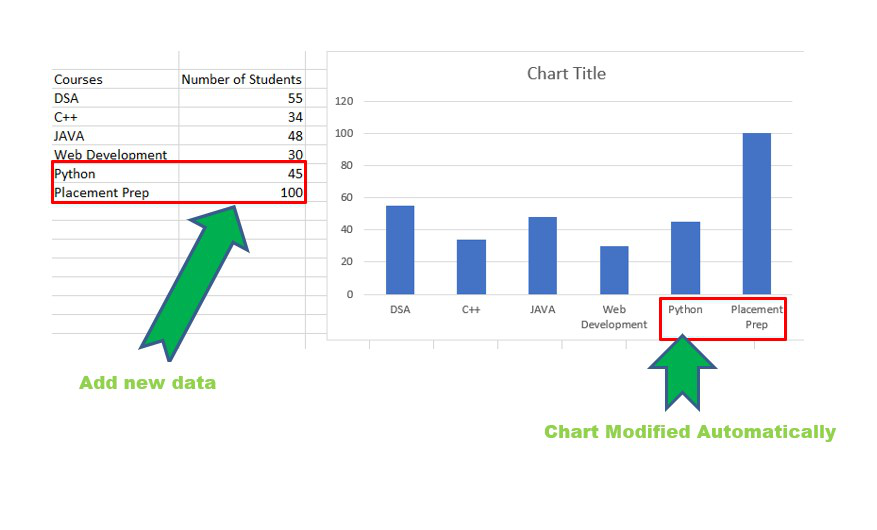Whether you're preparing for your first job interview or aiming to upskill in this ever-evolving tech landscape, GeeksforGeeks Courses are your key to success. We provide top-quality content at affordable prices, all geared towards accelerating your growth in a time-bound manner. Join the millions we've already empowered, and we're here to do the same for you. Don't miss out - check it out now!

Previous
Next